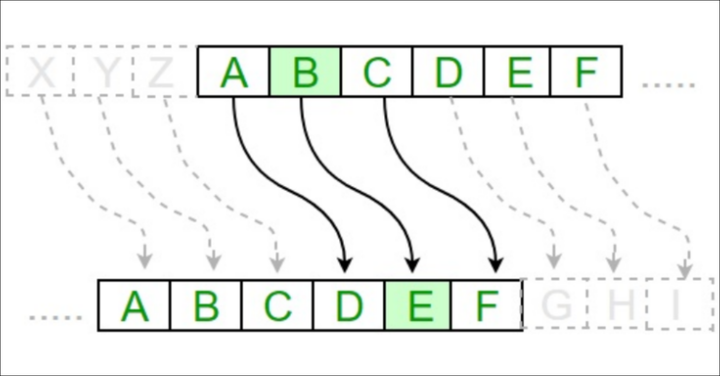# Algorithm of Caesar Cipher

Caesar Cipher Technique is a simple and easy method of encryption techniqueThe key to the Caesar Cipher will be a number from 1 to 26.

The Caesar Cipher was one of the earliest ciphers ever invented. In this cipher, you encrypt a message by taking each letter in the message (in cryptography, these letters are called symbols because they can be letters, numbers, or any other sign) and replacing it with a “shifted” letter.

• Caesar Cipher Technique is a simple and easy method of encryption technique.
• It is a simple type of substitution cipher.
• Each letter of plain text is replaced by a letter with some fixed number of positions down with the alphabet.

We also show brute force to Caesar Cipher. Brute force is the technique of trying every possible key until you find the correct one. Because there are only 26 possible keys, it would be easy for a cryptanalyst to write a hacking program than decrypts with every possible key. Then they could look for the key that decrypts to plain English. Let’s add a brute force feature to the program.

### Code


MAX_KEY_SIZE = 26

def getMode():
while True:
print('Do you wish to encrypt or decrypt or brute force a message?')
mode = input().lower()
if mode in 'encrypt e decrypt d brute b'.split():
return mode
else:
print('Enter either "encrypt" or "e" or "decrypt" or "d" or "brute" or "b".')

def getMessage():
return input()

def getKey():
key = 0
while True:
print('Enter the key number (1-%s)' % (MAX_KEY_SIZE))
key = int(input())
if (key >= 1 and key <= MAX_KEY_SIZE):
return key

def getTranslatedMessage(mode, message, key):
if mode == 'd':
key = -key
translated = ''

for symbol in message:
if symbol.isalpha():
num = ord(symbol)
num += key

if symbol.isupper():
if num > ord('Z'):
num -= 26
elif num < ord('A'):
num += 26
elif symbol.islower():
if num > ord('z'):
num -= 26
elif num < ord('a'):
num += 26

translated += chr(num)
else:
translated += symbol
return translated

mode = getMode()
message = getMessage()
if mode != 'b':
key = getKey()

if mode != 'b':
print(getTranslatedMessage(mode, message, key))
else:
for key in range(1, MAX_KEY_SIZE + 1):
print(key, getTranslatedMessage('decrypt', message, key))



### Output

Do you wish to encrypt or decrypt a message?
encrypt
Sergio Mendonca
Enter the key number (1-26)
3